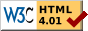## Dokumentation zu: sort_array(E)``` SYNOPSIS mixed * sort_array(mixed *arr, string wrong_order) mixed * sort_array(mixed *arr, string wrong_order, object|string ob) mixed * sort_array(mixed *arr, string wrong_order, object|string ob , mixed extra...) mixed * sort_array(mixed *arr, closure cl) mixed * sort_array(mixed *arr, closure cl, mixed extra...) DESCRIPTION Sort the copy either by the ordering function ob->wrong_order(a, b), or by the closure expression . Usually a shallow copy of is made first and the sorted copy is returned as result. However, if is given as a reference, no copy will be made and will be sorted in-place. If the argument equals 0, the result is also 0. is the object in which the ordering function is called and may be given as object or by its filename. If is omitted or neither a string nor an object, it defaults to this_object(). The elements from the array to be sorted are passed in pairs to the function as arguments, followed by the arguments if any. The function should return a positive number if the elements are in the wrong order. It should return 0 or a negative number if the elements are in the correct order. EXAMPLES To sort an array arr = ({ 3, 8, 1, 3 }) in ascending order with the help of the ordering function int is_greater (int a, int b) { return a > b; } the following uses of sort_array() are equivalent: arr = sort_array(arr, "is_greater", this_object()) arr = sort_array(arr, "is_greater") arr = sort_array(arr, #'is_greater) arr = sort_array(arr, #'>) (this is the preferred variant :-) arr = sort_array(arr, lambda(({'a, 'b}), ({#'>, 'a, 'b}))) If no implicit shallow copy of should be made, pass as reference: sort_array(&arr, #'>) A more complicated example is to sort the array arr = ({ ({ "foo", 3 }), ({ "quux", 1 }), ... }) in ascending order by the second element of each subarray. For this, the ordering function should be like int is_greater (mixed *a, mixed *b) { return a > b; } HISTORY LDMud 3.2.8 added the support of extra arguments. LDMud 3.3.720 added the support of references to sort in-place. SEE ALSO transpose_array(E), filter(E), map(E), alists(LPC) ``` Die Seite ist auch in Deutsch vorhanden.

Start » Magierhandbuch » Docu » Efun » Sort_array Letzte Generierung: 25.04.2021, 01:58mud@wl.mud.de